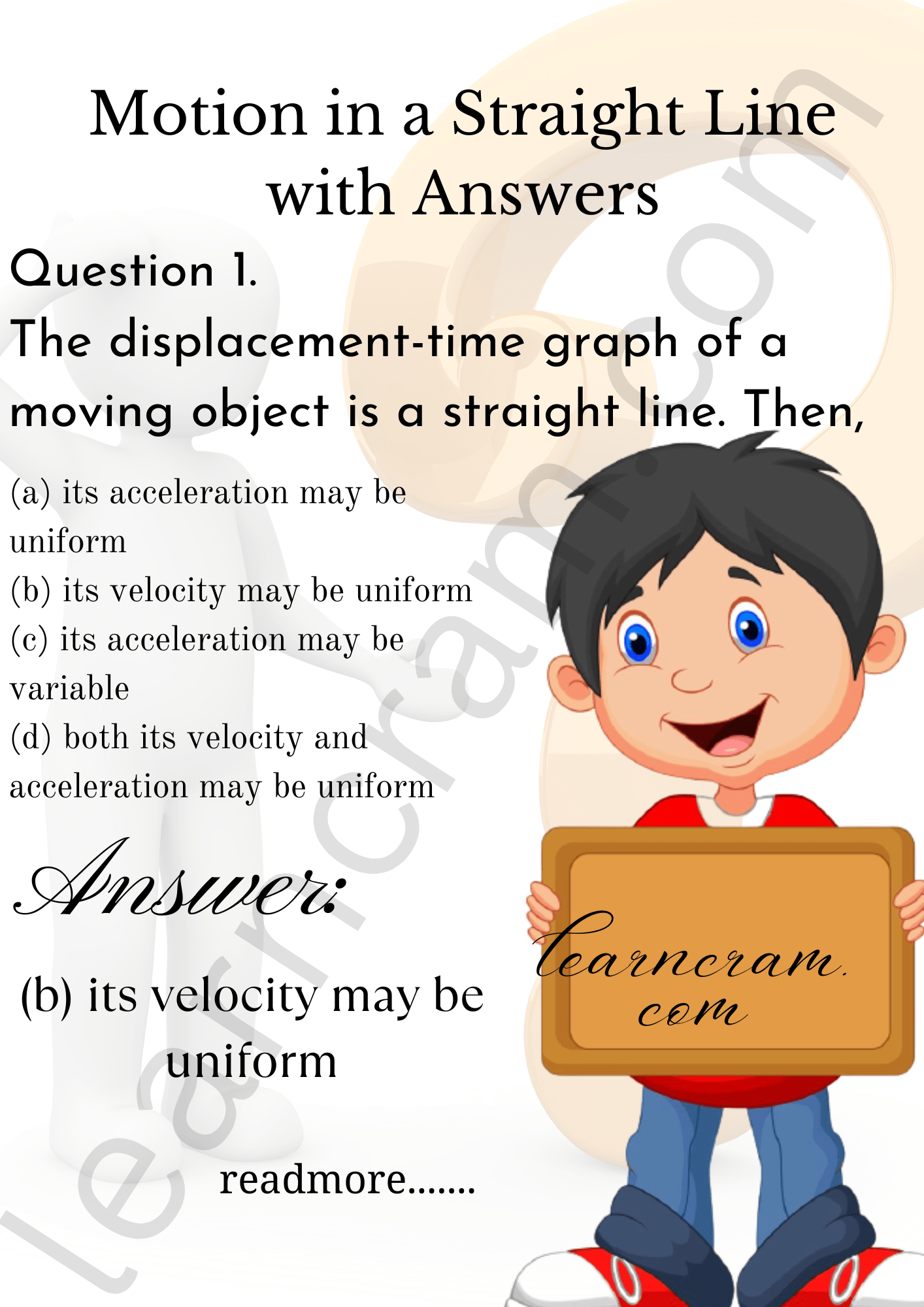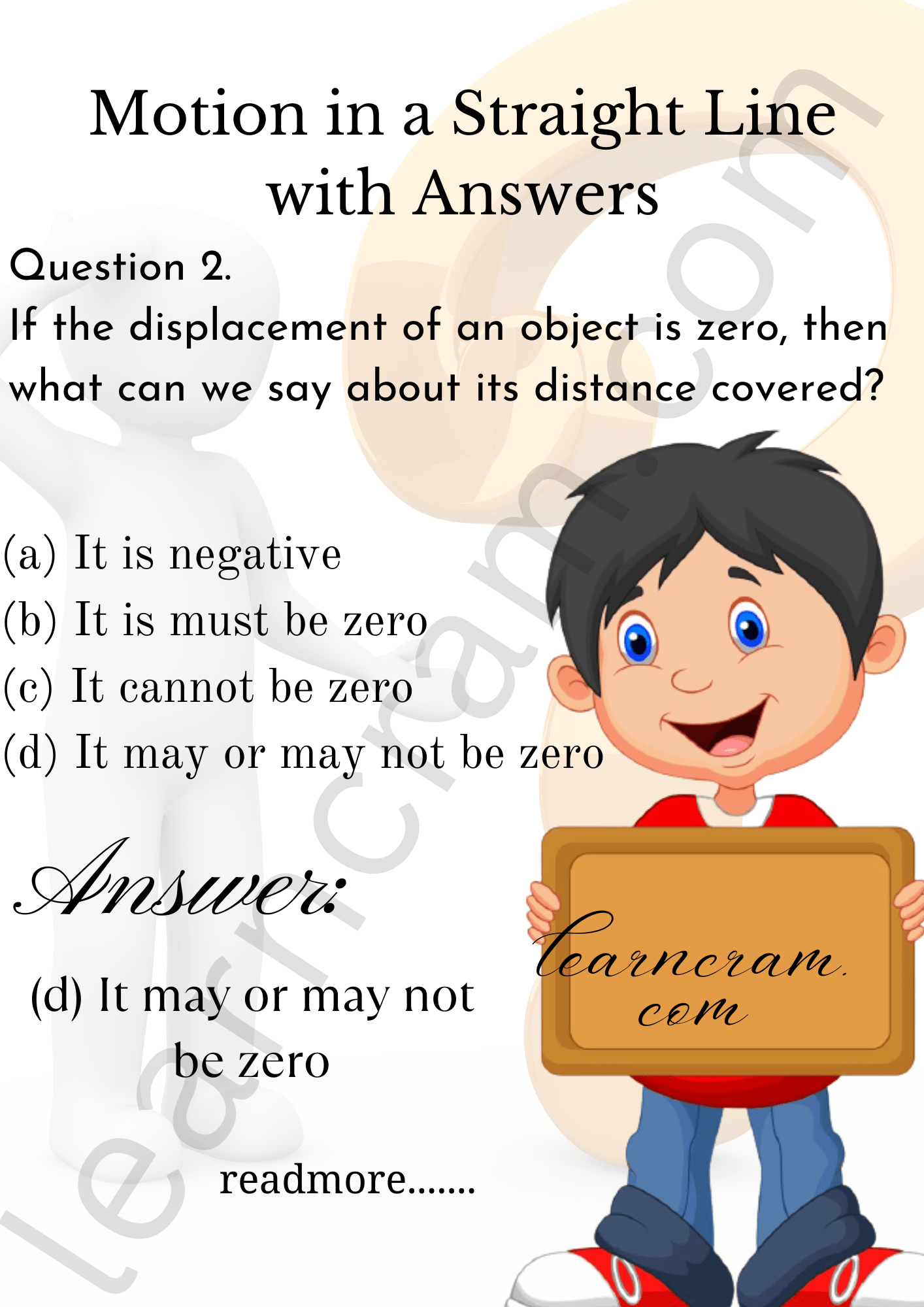# MCQ Questions for Class 11 Physics Chapter 3 Motion in a Straight Line with Answers

We have compiled the NCERT MCQ Questions for Class 11 Physics Chapter 3 Motion in a Straight Line with Answers Pdf free download covering the entire syllabus. Practice MCQ Questions for Class 11 Physics with Answers on a daily basis and score well in exams. Refer to the Motion in a Straight Line Class 11 MCQs Questions with Answers here along with a detailed explanation.

## Motion in a Straight Line Class 11 MCQs Questions with Answers

Multiple Choice Questions

Question 1.
The displacement-time graph of a moving object is a straight line. Then,
(a) its acceleration may be uniform
(b) its velocity may be uniform
(c) its acceleration may be variable
(d) both its velocity and acceleration may be uniform

Answer: (b) its velocity may be uniformQuestion 2.
If the displacement of an object is zero, then what can we say about its distance covered?
(a) It is negative
(b) It is must be zero
(c) It cannot be zero
(d) It may or may not be zero

Answer: (d) It may or may not be zeroQuestion 3.
Which of the following changes when a particle is moving with uniform velocity?
(a) Speed
(6) Velocity
(c) Acceleration
(d) Position vector

Question 4.
The distance travelled by an object is directly proportional to the time taken. Its acceleration
(a) increases
(b) decreases
(c) becomes zero
(d) remains constant

Question 5.
The distance travelled by an object is directly proportional to the time taken. Its speed
(a) increases
(b) decreases
(c) becomes zero
(d) remains constant

Question 6.
A particle is moving with a constant speed along straight line path. A force is not required to
(a) change its direction
(b) increase its speed
(c) decrease its momentum
(d) keep it moving with uniform velocity

Answer: (d) keep it moving with uniform velocity

Question 7.
If the velocity-time graph of an object is a straight line sloping downwards, the body has
(a) zero acceleration
(b) positive acceleration
(c) constant acceleration
(d) negative acceleration

Question 8.
When a body is dropped from a tower, then there is an increase in its
(a) mass
(b) velocity
(c) acceleration
(d) potential energy

Question 9.
If s represents distance and S represents displacement, then $$\frac {|S|}{s}$$ is.
(a) > 1
(b) < 1
(c) = 1
(d) ≤ 1

Question 10.
The velocity time graph of motion of an object starting from rest with uniform acceleration is a straight line
(a) parallel to time axis
(b) parallel to velocity axis
(c) passing through origin
(d) none of the above

Question 11.
If the displacement-time graph of an object is parallel to the time-axis, then it represents that the object is :
(a) at rest
(b) in uniform motion
(c) in acceleration motion
(d) none of the above

Question 12.
If the displacement of a given body is found to be directly proportional to the cube of the time elapsed, then the magnitude of the acceleration of the body is
(a) zero
(b) constant but not zero
(c) increasing with time
(d) decreasing with time

Question 13.
The acceleration of a moving object can be found from
(a) area under displacement-time graph
(b) slope of displacement-time graph
(c) area under velocity-time graph
(d) slope of velocity-time graph

Answer: (d) slope of velocity-time graph

Question 14.
The total vertical distance covered uy a freely falling body in a given time is directly proportional to
(a) time
(b) square of time
(c) square of acceleration due to gravity
(d) product of the time and acceleration due to gravity

Question 15.
A simple pendulum hangs from the roof of a train. The string is inclined towards the rear of the train. What is the nature of motion of the train?
(a) Uniform
(b) Accelerated
(c) Retarded
(d) At rest

Question 16.
A bucket is placed in the open where the rain is falling vertically. If a wind begins to blow at double the velocity of the rain, how will be the rate of filling of the bucket change?
(a) Remains unchanged
(b) Doubled
(c) Halved
(d) Becomes four times

Question 17.
Two particles start from rest simultaneously and are equally accelerated. Throughout the motion, the relative velocity of one w.r.t. other is :
(а) zero
(b) non-zero and directed parallel to acceleration
(c) non-zero and directed opposite to acceleration
(d) directed perpendicular to the acceleration

Question 18.
The relative velocity of a particle moving with a velocity v w.r.t. itself is
(a) v
(b) -v
(c) zero
(d) none of the above

Question 19.
A stone is thrown upward and it rises to a height of 100 m. The relative velocity of the stone w.r.t. the Earth will be maximum at:
(a) the ground
(b) a height of 50 m
(c) a height of 5 m
(d) the highest point

Question 20.
Two particles are moving with velocities v1 and v2. Their relative velocity is maximum when the angle between their velocities
(a) zero
(b) $$\frac {π}{2}$$
(c) π
(d) $$\frac {π}{4}$$

Fill in the blanks

Question 1.
The distances travelled by a body falling freely from rest in the first, second and third seconds are in the ratio …………………

Answer: 1 : 3 : 5.

Question 2.
The slope of the velocity-time graph for accelerated motion is …………………

Question 3.
The velocity-time graph of the body at rest is …………………

Answer: a straight line parallel to the time axis.

Question 4.
A moving body is covering distances in proportion to the square of the time along a straight line, its acceleration is …………………

Question 5.
The area under v-t graph for uniform motion gives …………………

Answer: the distance covered by the object.

Question 6.
If the distance covered by a particle is zero, then its displacement …………………

Question 7.
If the displacement of a particle is zero, then the distance covered by it …………………

Answer: may or may not be zero.

Question 8.
A car travels a distance s on a straight road in two hours and then returns to the starting point in the next three hours, its average velocity will be …………………

Question 9.
A particle moves along a straight line path. After sometime it comes to rest. The motion is with constant acceleration whose direction with respect to the direction of velocity is …………………

Question 10.
In a one dimensional motion with constant acceleration, the time rate of change of speed is independent of …………………

True/False Type Questions

Question 1.
Displacement is independent of the choice of origin of the axis.

Question 2.
Displacement may or may not be equal to the distance travelled.

Question 3.
When a particle returns to its starting point, its displacement is zero.

Question 4.
A positive acceleration always corresponds to the speeding up and a negative acceleration always corresponds to the speeding down.

Question 5.
Speed can never be negative.

Question 6.
If the velocity of a particle is zero at an instant, its acceleration should also be zero at that instant.

Question 7.
When the particle returns to the starting point, its average velocity is zero but average speed is not zero.

Question 8.
Displacement does not tell the nature of the actual motion of a particle between the points.

Question 9.
non-zero acceleration without having varying speed.

Question 10.
non-zero acceleration without having vary ing velocity.

Question 11.
varying speed without having varying velocity.

Question 12.
varying velocity without having varying speed.

Question 13.
If the velocity is zero at any instant, the acceleration should also be zero at that instant.

Question 14.
If the velocity is zero for a time interval, the acceleration is zero at any instant within the time interval.

Question 15.
If the position and velocity have opposite signs, the particle is moving towards the origin.

Question 16.
If the velocity and acceleration have opposite signs, the object is slowing down.

Question 17.
It is possible to have a situation in which the speed of the particle is never zero but the average speed in an interval is zero.

Question 18.
It is possible to have a situation in which the speed of a particle is always zero but the average speed is not zero.

Question 19.
The magnitude of the velocity of a particle is equal to its speed.

Question 20.
The magnitude of average velocity in an interval is equal to its average speed in that interval.

Question 21.
square of time

Question 22.
time

Question 23.
square of acceleration due to gravity

Question 24.
product of acceleration due to gravity and time.

Question 25.
Displacement is always positive.

Question 26.
Displacement has both magnitude and direction.

Question 27.
Displacement can be represented geometrically.

Question 28.
Magnitude of displacement is equal to the shortest distance between the initial and final positions of the object.

Question 29.
The motion is always in the same direction.

Question 30.
The motion is along a straight line path.

Question 31.
Average velocity is equal to the instantaneous velocity.

Question 32.
Magnitude of displacement < distance covered.

Question 33.
Its speed is zero.

Question 34.
Its acceleration is zero.

Question 35.
Its acceleration is opposite to the velocity.

Question 36.
Its speed may be variable.

If the distance covered by a particle is zero, then which statement is true/false.
Question 37.
It is negative.

Question 38.
It must be zero.

Question 39.
It cannot be zero.

Question 40.
It may or may not be zero.